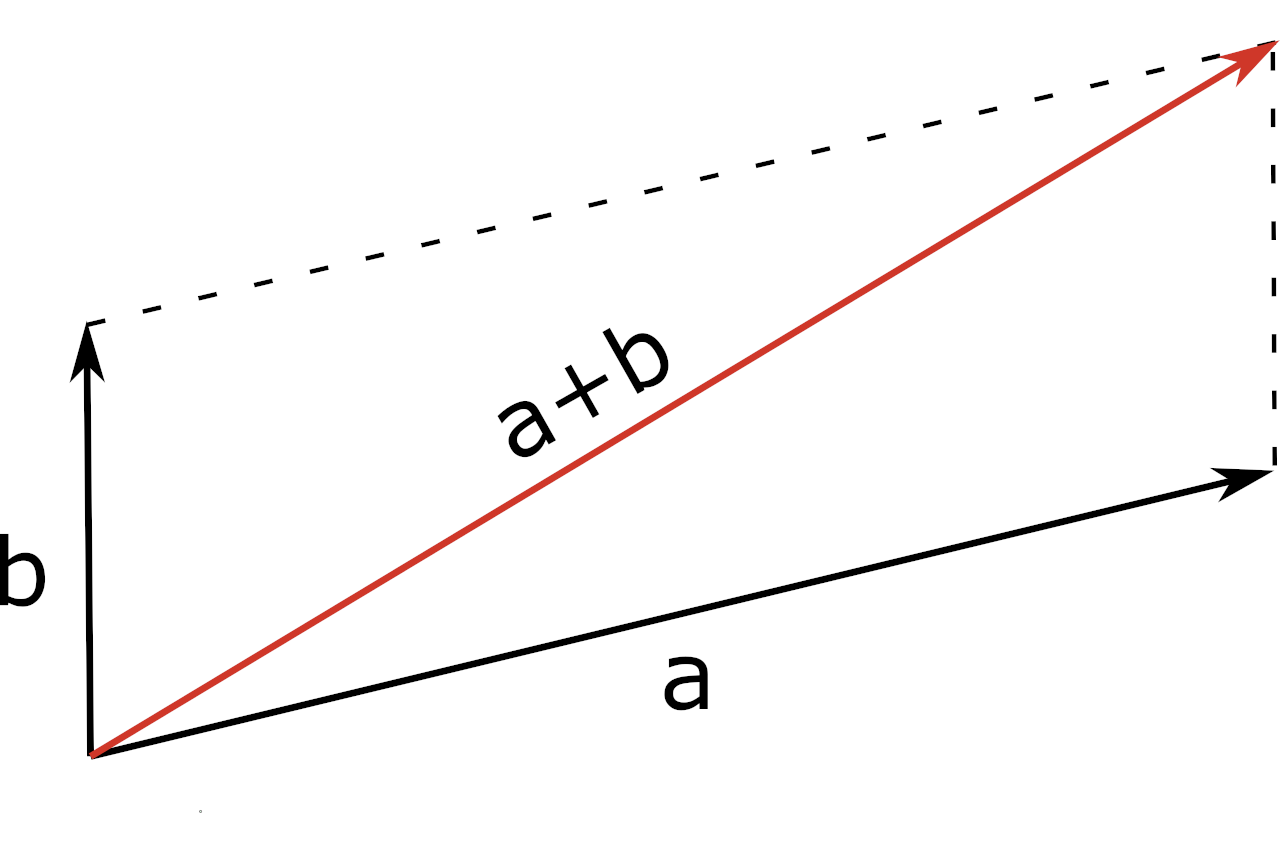Add two vectors a and b to find resultant vector a + b using the calculator below.

Vector a
Vector b
Vector a
Vector b

## Vector (a + b):

### Steps to Solve

#### Use the Vector Addition Formula

a + b = ({xa + xb}, {ya + yb}, {za + zb})

#### Substitute Values and Solve

Enter vectors a & b above to see the solution here

Learn how we calculated this below

In linear algebra, vectors represent an ordered sequence of numbers. You can add two or more vectors by adding the corresponding components together.

The sum of two vectors is referred to as the resultant vector. You can also subtract vectors in a similar fashion.

So, the vector addition formula is as follows:

a + b = ({xa + xb}, {ya + yb}, {za + zb})

Thus, vector a + b is equal to the sum of the x coordinates of each vector, the sum of the y coordinates of each vector, and the sum of the z coordinates of each vector.

## Parallelogram Method

You can also add vectors a and b using the parallelogram method.

Using this method, place the two vectors at the same initial point. Then, complete the parallelogram by drawing two additional lines.The diagonal of the parallelogram is equal to the resultant vector a + b.

You can also use our cross product calculator or dot product calculator to multiply vectors.